# How to add a Calculated Field

## This article will go over how to add a Calculated Field to a table and will provide some common formulas.

Casebook allows you to define new fields in the data set, named calculated fields. These fields are created by using expressions (formulas). An expression can be a combination of existing field(s), constant values and: predefined functions, and/or.

### Instructions for adding a calculated field:

1. From the Report Builder, Select the + Icon in Fields Section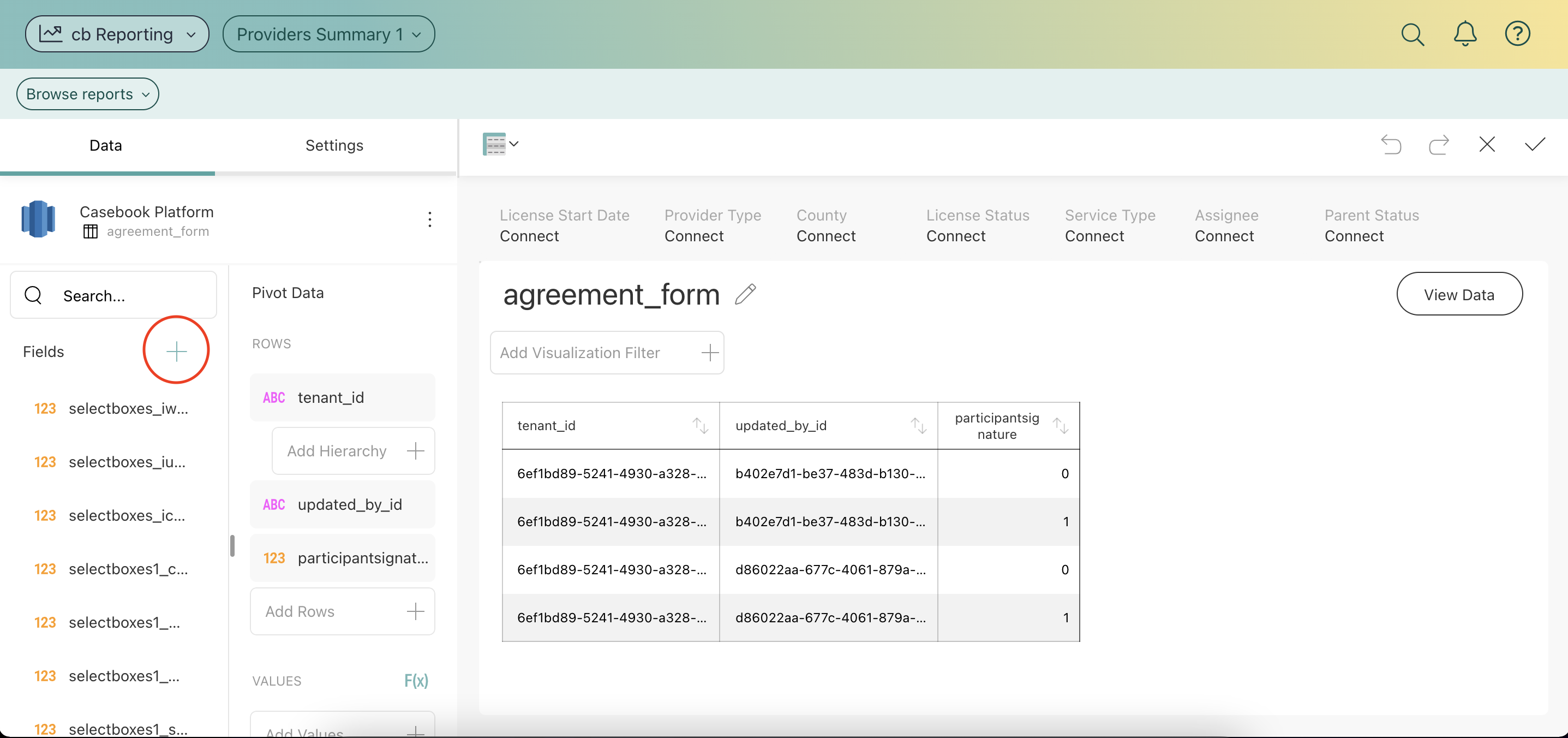2. Select Calculated Field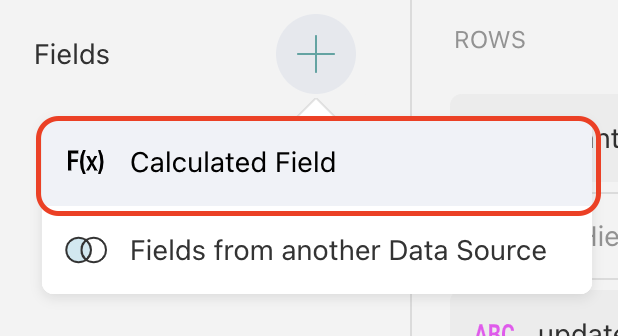3. Name the Calculated Field and select Done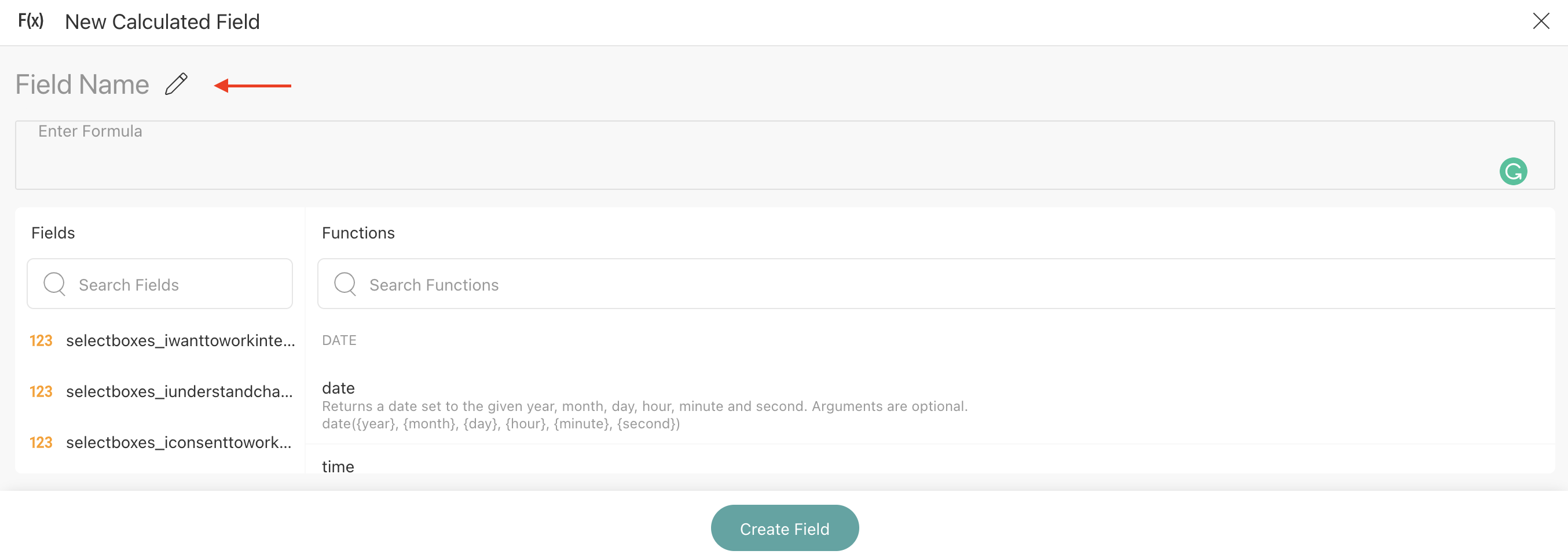4. Enter in a formula or copy and paste the formula that you wish to use and select Create Field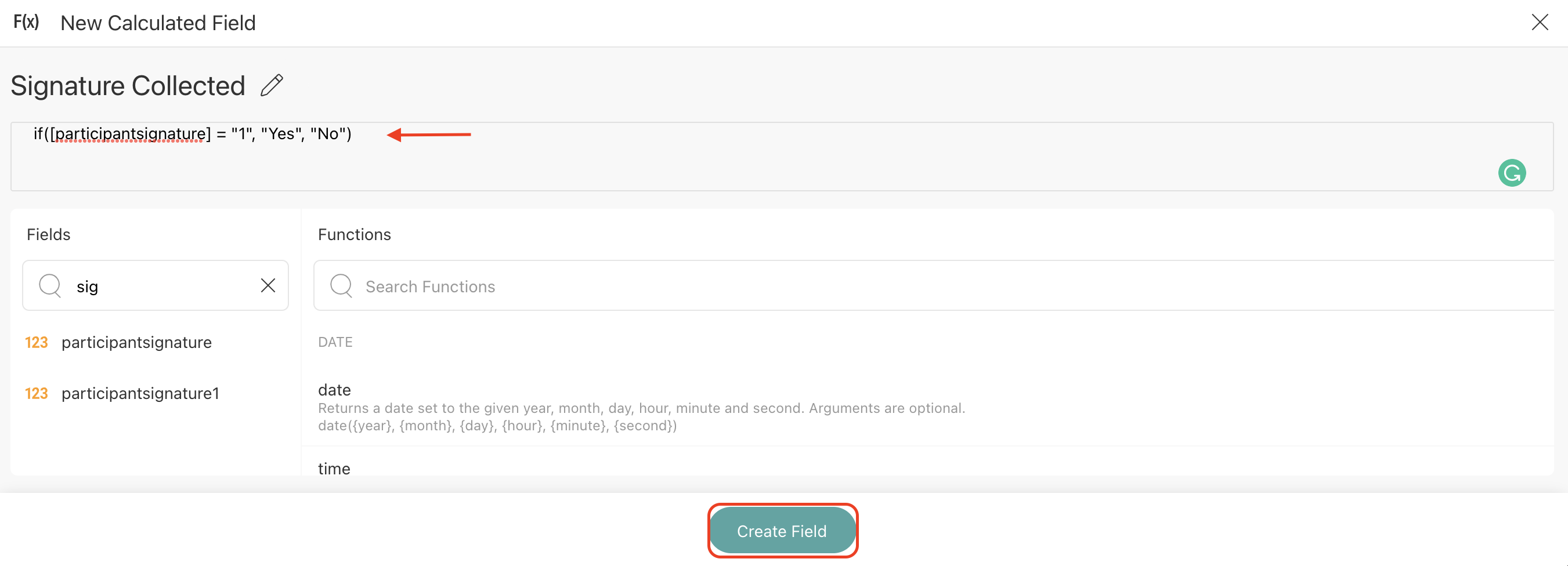5. The new Calculated Field will populate at the bottom of the Fields list on the left-hand side of the Report Builder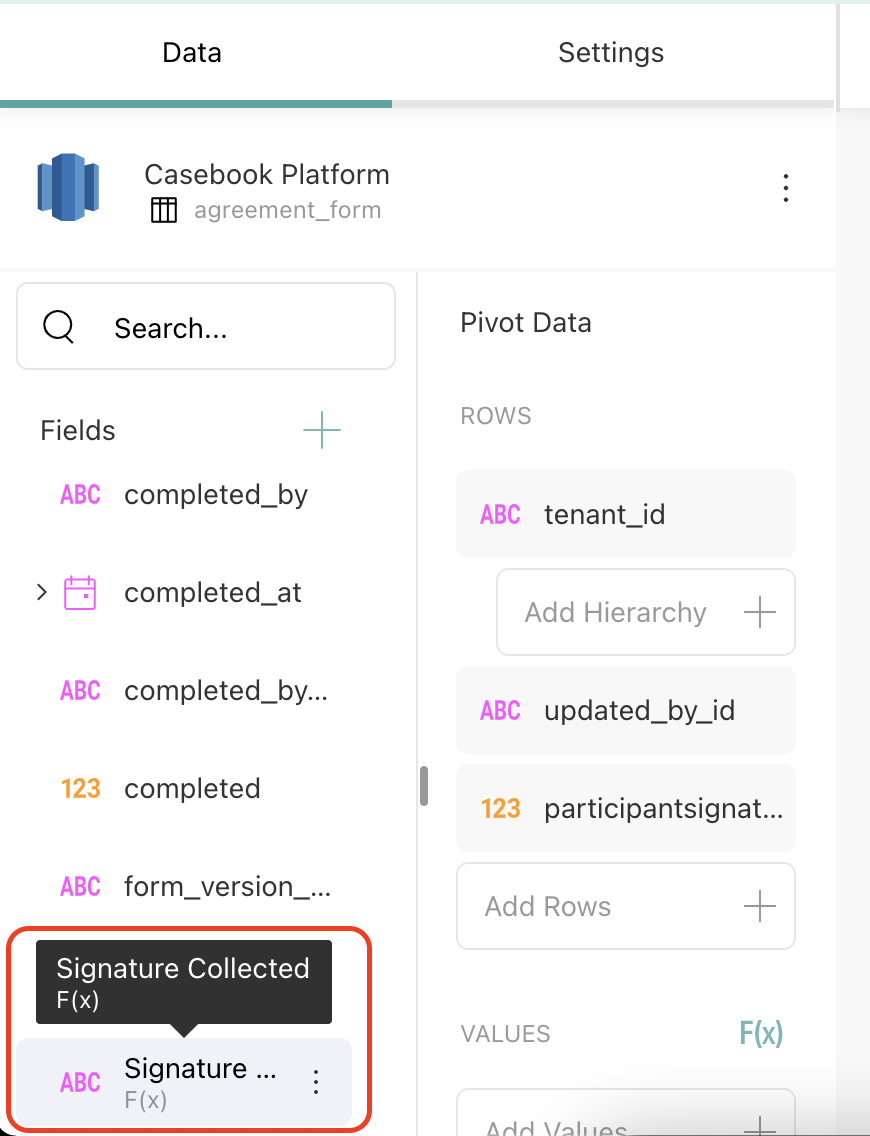To see these steps in action, check out the video below:### Common Formulas to Copy / Paste:

• Find Cases Without a Person
if(isempty(person_id]), “Missing Data”, “Data is entered”)
• Find People Without a Case
if(isempty(case_id]), “Missing Data”, “Data is entered”)
• Find Children
if(age < 18, "Under 18", "Over 18")
• Attended Training
if([service_name] = “training', “Yes”, “No”) or if ([service_name] = ‘training’, “Attended training”, “Did not attend training”)
• Fiscal Year 2021
if (isempty([service_end_date]), if([service_start_date] > date(2021,06,30), if([service_start_date] < date(2022, 07, 01), "Fiscal 2021", "Not Fiscal 2021"), "Fiscal 2021"), if([service_end_date] > date(2021,06,30), if([service_end_date] < date(2022, 07, 01), "Fiscal 2021", if([service_start_date] > date(2021,06,30), if([service_start_date] < date(2022, 07, 01), "Fiscal 2021", "Not Fiscal 2021"), "Fiscal 2021")), "Not Fiscal 2021"))
• Q1 Fiscal Year 2021
if(isempty([service_end_date]), if(quarter([service_start_date]) = 3, if(year([service_start_date]) = 2021, "Fiscal Q1", "Not Fiscal Q1"), if (quarter(today()) = 3, if(year(today()) = 2021, "Fiscal Q1", "Not Fiscal Q1"), "Not Fiscal Q1")),if(quarter([service_start_date]) = 3, if(year([service_start_date]) = 2021, "Fiscal Q1", "Not Fiscal Q1"), if(quarter([service_end_date]) = 3, if(year([service_end_date]) <= 2022, "Fiscal Q1", "Not Fiscal Q1"), "Not Fiscal Q1")))
• Q2 Fiscal Year 2021
if(isempty([service_end_date]), if(quarter([service_start_date]) =< 4, if(year([service_start_date]) = 2021, "Fiscal Q2", "Not Fiscal Q2"), if (quarter(today()) = 4, if(year(today()) = 2021, "Fiscal Q2", "Not Fiscal Q2"), "Not Fiscal Q2")),if(quarter([service_start_date]) = 4, if(year([service_start_date]) = 2021, "Fiscal Q2", "Not Fiscal Q2"), if(quarter([service_end_date]) = 4, if(year([service_start_date]) = 2021, "Fiscal Q2", "Not Fiscal Q2"), "Not Fiscal Q2")))
• Q3 Fiscal Year 2021
if(isempty([service_end_date]), if(quarter([service_start_date]) = 1, if(year([service_start_date]) = 2022, "Fiscal Q3", "Not Fiscal Q3"), if (quarter(today()) = 1, if(year(today()) = 2022, "Fiscal Q3", "Not Fiscal Q3"), "Not Fiscal Q3")),if(quarter([service_start_date]) = 1, if(year([service_start_date]) = 2022, "Fiscal Q3", "Not Fiscal Q3"), if(quarter([service_end_date]) = 1, if(year([service_end_date]) = 2022, "Fiscal Q3", "Not Fiscal Q3"), "Not Fiscal Q3")))
• Q4 Fiscal Year 2021
if(isempty([service_end_date]), if(quarter([service_start_date]) =< 2, if(year([service_start_date]) = 2022, "Fiscal Q4", "Not Fiscal Q4"), if (quarter(today()) = 2, if(year([service_start_date]) = 2022, "Fiscal Q4", "Not Fiscal Q4"), "Not Fiscal Q4")),if(quarter([service_start_date]) = 2, if(year([service_start_date]) = 2022, "Fiscal Q4", "Not Fiscal Q4"), if(quarter([service_end_date]) = 2, if(year([service_end_date]) = 2022, "Fiscal Q4", "Not Fiscal Q4"), "Not Fiscal Q4")))
• Fiscal Year 2022
if(and([service_start_date]> date(2021,12,31), [service_start_date] < date(2023,1,1)),"Fiscal 2022",if(isempty([service_end_date]), if(and([service_start_date] < date(2023,1,1), today()> date(2021,12,31)), "Fiscal 2022", "Not Fiscal 2022"),if(and([service_start_date] < date(2023,1,1), [service_end_date]> date(2021,12,31)), "Fiscal 2022", "Not Fiscal 2022")))
• Q1 Fiscal Year 2022
if(isempty([service_end_date]),if(and([service_start_date] < date(2022,4,1),(today() > date(2021,12,31))),"Fiscal Q1","Not Fiscal Q1"),if(and([service_start_date] < date(2022,4,1),([service_end_date] > date(2021,12,31))),"Fiscal Q1","Not Fiscal Q1"))
• Q2 Fiscal Year 2022
if(isempty([service_end_date]),if(and([service_start_date] < date(2022,7,1),(today() > date(2022,3,31))),"Fiscal Q2","Not Fiscal Q2"),if(and([service_start_date] < date(2022,7,1),([service_end_date] > date(2022,3,31))),"Fiscal Q2","Not Fiscal Q2"))
• Q3 Fiscal Year 2022
if(isempty([service_end_date]),if(and([service_start_date] < date(2022,10,1),(today() > date(2022,6,30))),"Fiscal Q3","Not Fiscal Q3"),if(and([service_start_date] < date(2022,10,1),([service_end_date] > date(2022,6,30))),"Fiscal Q3","Not Fiscal Q3"))
• Q4 Fiscal Year 2022
if(isempty([service_end_date]),if(and([service_start_date] < date(2023,1,1),(today() > date(2022,9,30))),"Fiscal Q4","Not Fiscal Q4"),if(and([service_start_date] < date(2023,1,1),([service_end_date] > date(2022,9,30))),"Fiscal Q4","Not Fiscal Q4"))